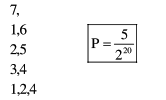# Let the function`
Question:

Let $S=\{1,2, \ldots \ldots, 20\}$. A subset $B$ of $S$ is said to be "nice", if the sum of the elements of B is 203 . Then the probability that a randomly chosen subset of $S$ is "nice" is :-

1. $\frac{6}{2^{20}}$

2. $\frac{5}{2^{20}}$

3. $\frac{4}{2^{20}}$

4. $\frac{7}{2^{20}}$

Correct Option: , 2

Solution: